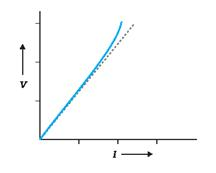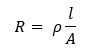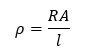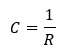0
30

# Ohm’s Law

The electric current flowing through a substance is proportional to the voltage across its ends, i.e.,

V ∝ I

V = RI,

where is called the resistance of the substance.The unit of resistance is ohm: 1Ω = 1 V A-1.

The resistance R not only depends on the material of the conductor but also on the dimensions of the conductor.

### Ohm’s Law,Unit: Current I in ampere (A) , potential difference V is in volt (V)

### Resistance of a uniform conductor,Unit: ohm (Ω)

### Resistivity or specific resistance,Unit: Ωm

### Conductance,Unit: ohm-1 or mho or Siemens(S)

Unit: Ω-1m-1

Unit: Am-2